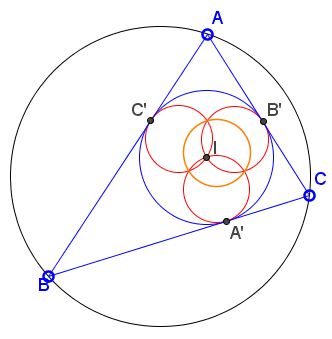# Inversion in the Incircle What Is It About? A Mathematical Droodle

Explanation### Inversion in the Incircle

The applet suggests the following theorem [Mathematical Gems II]:

The inverse images of the side lines of a triangle in its incircle are three circles of equal radii that concur at the incenter. The circle through their second points of intersection is none other than the inverse image of the circumcircle. It has the same radius.R. Honsberger credits Arnold Emch (1916) with the following observation.

For a given $\Delta ABC,$ we are interested in the inversion in its incircle. The side lines (being tangent to the incircle) invert into the circles that pass through the incenter $I$ and still tangent to the side lines at the same points $A',$ $B',$ $C'$ of tangency, as the side lines. These circles have $IA',$ $IB',\;$ and $IC'$ as their diameters. The three are therefore equal.

Their second points of intersection correspond by the inversion to the vertices of $\Delta ABC.\;$ It follows that the circle through these three points is the inverse image of the circumcircle of $\Delta ABC.\;$ As we know, it has the same diameter as the other three circles, viz., $r$ - the inradius of $\Delta ABC.\;$ We have obtained a nice result:

Under inversion in the incircle of a triangle, the side lines and the circumcircle are carried into four equal circles with the common diameter equal to the inradius of $\Delta ABC. ### References 1. R. Honsberger, Mathematical Gems II, MAA, 1976, pp. 20-21### Corollary Nathan Bowler has noticed that the above configuration yields a simple proof of the well known identity (yet another Euler's formula): (*)$OI^{2} = R^{2} - 2Rr,$where$R$and$r$are the circumradius and the inradius of$\Delta ABC.$Let$P$and$Q$be the two points of intersection of$OI$with the circumcircle, such that$IP = R - OI\;$and$IQ = R + OI.$Let$P'$and$Q'$be the inverses of$P$and$Q,\;$respectively. Then, since$P'Q'\;$is the diameter of the inverse image of the circumcircle,$IP' + IQ' = r.$Now we have$\begin{align} r&= IP' + IQ'\\ &= r^{2}/IP + r^{2}/IQ\\ &= r^{2}/(R - OI) + r^{2}/(R + OI)\\ &= r^{2}·2R / (R^{2} - OI^{2}), \end{align}$which simplifies to$R^{2} - OI^{2} = 2Rr.$The latter is equivalent to (*) and conventionally appears in the following form$\displaystyle\frac{1}{R - OI} + \frac{1}{R + OI} = \frac{1}{r}.$It is a curious fact that the inverse image of the circumcircle in the incircle is the 9-point circle of$\Delta A'B'C',$from which, too, we see that its diameter is equal to the inradius of$\Delta ABC.\$### Inversion - Introduction### Nine Point Circle### 3 Equal and Concurrent Circles